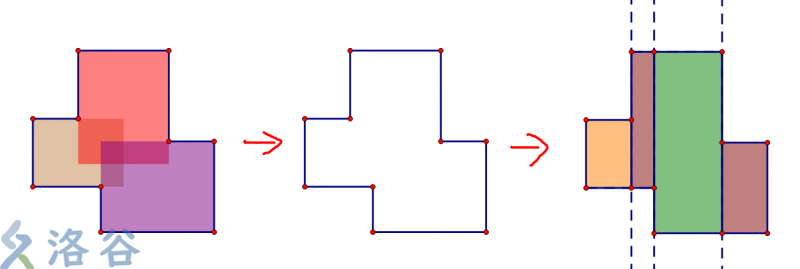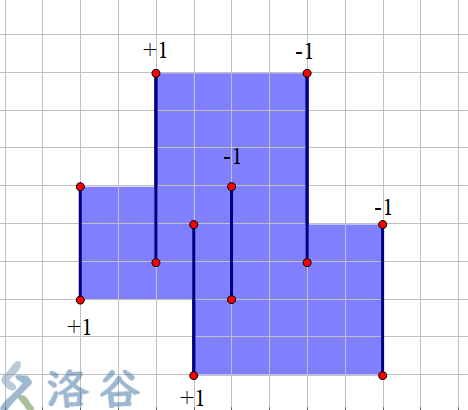# categories/刷题记录/共650篇文章zc
2019-12-21 19:47:00zc
2019-12-21 19:47:00

1. dp出结尾最小是多少

2. dp出字典序最大的方案

f_i表示以i结尾最靠近i的下标

f_i = max(j)|(num(f[j],j) < num(j+1,i))zc
2019-12-21 19:47:00zc
2019-12-21 19:47:00zc
2019-12-21 19:47:00zc
2019-12-21 19:47:00zc
2019-12-21 19:47:00zc
2019-12-21 19:47:00zc
2019-12-21 19:47:00zc
2019-12-21 19:47:00

 d(ij) = \sum_{x|i} \sum_{y|j}[gcd(x,y) = 1] 

n<m

 \sum_{i=1}^n \sum_{j=1}^m d(ij) \\ =\sum_{i=1}^n \sum_{j=1}^m \sum_{x|i} \sum_{y|j}[gcd(x,y) = 1] \\ =\sum\limits_{x=1}^n\sum\limits_{y=1}^m \left\lfloor\frac{n}{x}\right\rfloor \left\lfloor\frac{m}{y}\right\rfloor [\gcd(x,y)=1] 

x、y换成i、j

 \sum\limits_{i=1}^n\sum\limits_{j=1}^m \left\lfloor\frac{n}{i}\right\rfloor \left\lfloor\frac{m}{j}\right\rfloor[\gcd(i,j)=1] \\ =\sum\limits_{i=1}^n\sum\limits_{j=1}^m \left\lfloor\frac{n}{i}\right\rfloor \left\lfloor\frac{m}{j}\right\rfloor \sum_{k|gcd(i,j)}\mu(k) 

g(i)=\sum_{i=1}^n \left \lfloor \frac ni \right \rfloor

 ans=\sum_{k=1}^n\mu(k) g(\left \lfloor \frac nk \right \rfloor) g(\left \lfloor \frac mk \right \rfloor) \\ 

Search
search